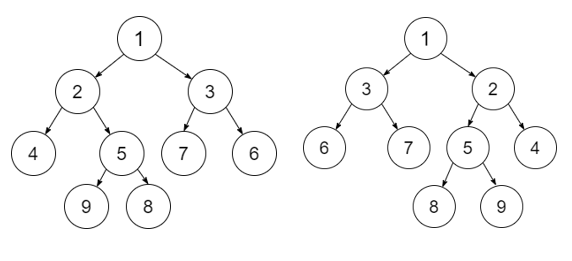# Check if a Tree is Isomorphic or not in C++

In a binary tree, each node contains two children, i.e., left child and right child. Let us suppose we have two binary trees and the task is to check if one of the tree can be obtained by flipping another tree by left of it or not.

A Tree is Isomorphic if it can be obtained by flipping the other tree in its left side.

For Example

Input-1Output: Isomorphic

Explanation: The given Tree-2 can be obtained by flipping the Tree-1 in the left side, thus the Tree is isomorphic.

## Approach to Solve this Problem

A recursive approach to solve this particular problem is that a Boolean function will check for the root nodes of both trees. If the roots of both the trees are empty or NULL, then return True and recursively check if both the roots have the same data. We will then check recursively for the left and right nodes of the tree.

• Create nodes for two binary trees.
• A Boolean Function isIsomorphicTree(node*r1, node*r2) takes the roots of two trees and returns if the tree is Isomorphic or not.
• Initially if the tree is empty or not having any nodes in it, then return True.
• If the subtree rooted is not flipped and if both are flipped, then return True.

## Example

Live Demo

#include<bits/stdc++.h>
using namespace std;
struct treenode {
int data;
treenode * left;
treenode * right;
};
struct treenode * createNode(int d) {
struct treenode * root = new treenode;
root -> data = d;
root -> left = NULL;
root -> right = NULL;
return root;
}
bool isIsomorphicTree(treenode * r1, treenode * r2) {
if (r1 == NULL and r2 == NULL) {
return true;
}
if (r1 == NULL or r2 == NULL) {
return false;
}
return (r1 -> data == r2 -> data &amp;&amp; ((isIsomorphicTree(r1 -> left, r2 -> right) &amp;&amp;       isIsomorphicTree(r1 -> right, r2 -> left)) || (isIsomorphicTree(r1 -> left, r2 -> left) &amp;&amp; isIsomorphicTree(r1 -> right, r2 -> right))));
}
int main() {
struct treenode * r1 = createNode(1);
r1 -> left = createNode(2);
r1 -> right = createNode(3);
r1 -> left -> left = createNode(4);
r1 -> left -> right = createNode(5);
r1 -> right -> left = createNode(6);
r1 -> left -> right -> left = createNode(7);
r1 -> left -> right -> right = createNode(8);
struct treenode * r2 = createNode(1);
r2 -> left = createNode(3);
r2 -> right = createNode(2);
r2 -> right -> left = createNode(4);
r2 -> right -> right = createNode(5);
r2 -> left -> right = createNode(6);
r2 -> right -> right -> left = createNode(8);
r2 -> right -> right -> right = createNode(7);
if (isIsomorphicTree(r1, r2)) {
cout << "Isomorphic" << endl;
} else {
cout << "Not an Isomorphic" << endl;
}
return 0;
}

Running the above code will generate the output as,

## Output

Isomorphic

Explanation: The given tree can be obtained by flipping the other tree by its left side, thus it is Isomorphic.